## raw data lettersIUCrDATA
ISSN: 2414-3146

## Accurate intensity integration in the twinned γ-form of o-nitro­aniline

aDepartment of Chemistry, Structural Biochemistry, Bijvoet Centre for Biomolecular Research, Faculty of Science, Utrecht University, Utrecht, The Netherlands
*Correspondence e-mail: l.m.j.kroon-batenburg@uu.nl

(Received 5 July 2022; accepted 3 November 2022; online 17 November 2022)

o-Nitro­aniline, C6H6N2O3, is known to be polymorphic. The α-form is probably amorphous, while the β- and γ-forms are crystalline. Difficulties with the unit-cell determination of the γ-form were reported as a consequence of twinning. In this paper, newly recorded diffraction data of the γ-form of o-nitro­aniline are described that were processed taking into account the two twin lattices. Data were partly deconvoluted and much better agreement was obtained in terms of R1 values and C—C bond precision. The availability of raw data and proper reprocessing using twin lattices is by far superior to efforts to de-twin processed structure factors.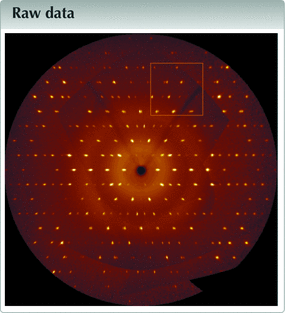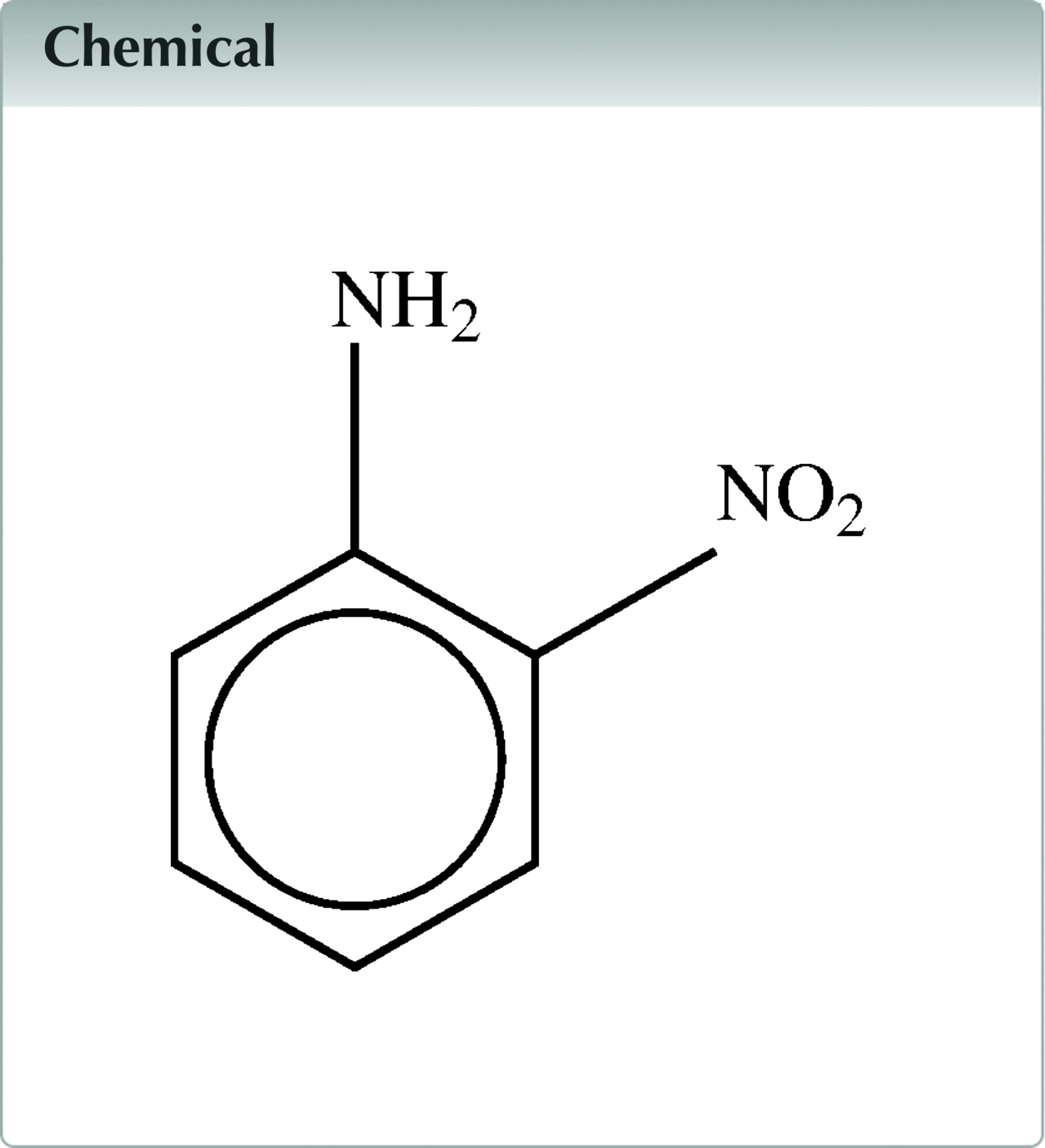### Introduction

o-Nitro­aniline is known to be polymorphic (Aakeröy et al., 1998a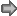,b). The α-form is probably amorphous, while the β- and γ-forms are crystalline. Difficulties with the unit-cell determination of the γ-form were reported as a consequence of twinning. The unit cell appears to be C-centered orthorhombic, but was determined to be a pseudo-merohedral monoclinic twin by Herbstein (1965), who also observed diffuse streaks along a*. Pseudo-orthorhombic twinning together with unusual extinctions was discussed by Dunitz (1964). While he assumed a twin obliquity of 0°, we here show an example where this is not exactly the case, i.e. the twin obliquity is 0.743°. The structure was determined before, supposedly from data of untwinned crystals, but the R1 values of 10.9, 7.01 and 7.98% remain large (Dhaneshwar et al., 1978; Nieger, 2007; Zych et al., 2007). In this paper we describe newly recorded diffraction data of the γ-form of o-nitro­aniline (I) and process these by taking into account the two twin lattices. We show that the availability of raw data and proper reprocessing using twin lattices is by far superior to efforts to de-twin processed structure factors.

### Data processing and refinement

Data were processed in two ways: by using a single lattice, ignoring the second lattice completely, and by using two twin lattices. EVAL software (Schreurs et al., 2010) was used for both, followed by SADABS/TWINABS (Krause et al., 2015; Sevvana et al., 2019) for scaling. Splitting of the radiation in Kα1 and Kα2 in a 2:1 ratio is taken into account in the EVAL model profiles for either lattice. The statistics for the two approaches are given in Table 1. Several indicators in the single-crystal data processing show that the crystal is not a single crystal. The first real sign of an alarm occurs when it comes to the space-group determination: the most likely space group is P21/a but systematic absences for the a-glide plane are clearly violated (reflection condition h0l; h = 2n). Structure refinement with SHELXL (Sheldrick, 2015) converges at high residuals R1[I > 2σ(I)] = 0.0787 and wR2(all refl.) = 0.2545 and the proposed weighting scheme is rather unusual. The two twin lattices are related by a twofold rotation about c, resulting in the twin matrix (−1 0 −1 / 0 −1 0 / 0 0 1) (see below). With only single-crystal structure factors it is still possible to use the knowledge of the twin matrix. Inclusion of this matrix in the SHELXL refinement assumes that the lattices overlap exactly and the obliquity would be 0°. In reality, not all reflections overlap and thus the refinement results improve only slightly {R1[I > 2σ(I)] = 0.0678 and wR2(all refl.) = 0.2390}. As a last resort, one can de-twin the merged data with TWINROTMAT in PLATON (Spek, 2020). This produces an HKLF5-type file for refinement in SHELXL in which each reflection is either overlapped or single (935 reflections are overlapping). The structure refinement improved to R1[I > 2σ(I)] = 0.0465 and wR2(all refl.) = 0.1332. As we will see below, it is a poor approach for resolving the twinning issue with processed data, clearly raw diffraction data are needed to reprocess with two lattices.

 Table 1Experimental details
 Raw data DOI https://doi.org/10.5281/zenodo.7193538 Data archive Zenodo Data format CBF Data collection Diffractometer Bruker Kappa APEXII Temperature (K) 150 Detector type APEXII CCD Radiation type Mo Kα Wavelength (Å) 0.71073 Beam center (mm) −30.401, −30.637 Detector axis −Z Detector distance (mm) 41 Swing angle (°) −21.52 Pixel size (µm) 0.12 × 0.12 No. of pixels 512 × 512 No. of scans 7 Exposure time per frame (s) 10 Scan axis Start angle, increment per frame (°) Scan range (°) No. of frames ϕ, −X (ω = 164.659°, κ = 46.226°) 74.659, −0.300 −360 1200 ω, −X (κ = −73.760°, ϕ = 10.746°) −169.393, −0.300 −118.2 394 ω, −X (κ = −73.760°, ϕ = −91.253°) −169.393, −0.300 −118.2 394 ω, −X (κ = 88.307°, ϕ = 160.033°) −157.189, −0.300 −82.2 274 ω, −X (κ = 88.307°, ϕ = 58.033°) −157.189, −0.300 −82.2 274 ω, −X (κ = −73.760°, ϕ = −40.253°) −169.393, −0.300 −118.2 394 ω, −X (κ = −73.760°, ϕ = 166.747°) −169.393, −0.300 −118.2 394 Crystal data Chemical formula C6H6N2O2 Mr 138.13 Crystal system, space group Monoclinic, P21/a a, b, c (Å) 15.2066 (5), 10.0938 (4), 8.3580 (2) β (°) 106.693 (3) V (Å3) 1228.82 (7) Z 8 μ (mm−1) 0.12 Crystal size (mm) 0.37 × 0.30 × 0.16 Data processing Twin Single lattice Absorption correction Multi-scan Multi-scan (TWINABS2012/1; Sevvana et al., 2019) (SADABS; Krause et al., 2015) Tmin, Tmax 0.683, 0.746 0.628, 0.746 No. of measured, independent and observed [I > 2σ(I)] reflections 26077, 2864, 2629 2145 overlapping and 842 single reflections and 123 systematic absences 24760, 2816, 2547 Rint 0.028 0.034 (sin θ/λ)max (Å−1) 0.655 0.655 Refinement No. of reflections 2864 2816 No. of parameters 198 197 H-atom treatment N—H refined freely; C—H refined with a riding model N—H refined freely; C—H refined with a riding model R[F2 > 2σ(F2)], wR(F2), S 0.0314, 0.0860, 1.085 0.0787, 0.2545, 1.154 Twin fraction BASF 0.2003 (10) Weighting scheme a = 0.0449, b = 0.2210 a = 0.0702, b = 6.2812 Δρmax, Δρmin (e Å−3) 0.23, −0.22 0.35, −0.36 Bond precision C—C (Å) 0.0017 0.0062

To show the advantage of proper processing we used two matrices. One twin component was clearly the largest and we processed the data with this lattice while including the second lattice as interfering in EVAL. Reflections are deconvoluted when the covariance of the overlapping intensities is below a given threshold. This led to 2145 overlapping and 842 single reflections and 123 systematic absences (Table 1). The agreement factors of the SHELXL refinement are much improved to R1[I > 2σ(I)] = 0.0314 and wR2(all refl.) = 0.0860 (Table 1) and the displacement ellipsoids of the two independent molecules are perfectly reasonable (Fig. 1).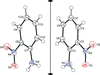Figure 1 Molecular structure of the two independent molecules of (I) in the crystal. Displacement ellipsoids are drawn at the 50% probability level. Hydrogen atoms are drawn as small spheres of arbitrary radii. The molecules are related by a non-crystallographic twofold axis approximately along a*.

The crystal structure has P21/a symmetry with two independent molecules, which are shown in Fig. 1. The molecules are connected by hydrogen bonds, forming two-dimensional layers in the bc plane (Fig. 2).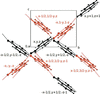Figure 2 Hydrogen-bonded layers in the monoclinic structure.

### Data description

Data were collected on our in-house APEXII diffractometer with Mo Kα radiation, with multiple scans (Table 1). In total 3324 images were recorded. The unit cell was determined with DIRAX (Duisenberg, 1992) and two lattices were found that could be transformed into each other with a nearly twofold rotation. Pseudo-orthorhombic twinning is characterized by a base-centered orthorhombic twin lattice derived from a monoclinic-P crystal lattice (Dunitz, 1964). The monoclinic P cell of (I) can be transformed to a near orthorhombic B lattice [the non-standard setting of the space group P21/a was chosen for compatibility with earlier literature (Herbstein, 1965); base-centered B-orthorhombic was chosen so as to leave b and c unchanged] with the following operation:giving cell parameters a′ = 29.1340 (10), b′ = 10.0938 (4), c′ = 8.3580 (2), Å, α′ = 90, β′ = 90.743 (3), γ′ = 90°. The c axis was chosen as the twofold twin rotation axis. Clearly we find a twin obliquity of 0.743 (3)° and a non-merohedral twin. As a consequence, the orthorhombic B lattices of the individual twin components do not exactly overlap. If one overlooks the twinning and indexes the spots as B-centered orthorhombic in space group B2212, the reflection conditions appear to be: hkl; h + l = 2n, 0k0; k = 2n, which is usual for the space group, and h00; h = 4n, 00 l; l = 4n. The latter two are non-space-group extinctions and exactly such observations are considered as a signal for pseudo-orthorhombic twinning of an underlying monoclinic lattice (Dunitz, 1964). Processing the data as single-component orthorhombic does not result in a structure solution, notably because the a glide plane is absent in B2212.

The twin rotation about the c-axis results in stacking faults of the hydrogen-bonded layers. In Fig. 3, the two domains and the twinning interface is shown. The second lattice was generated by 180° rotation around c followed by a translation over 1/2a, by which the two independent molecules are interchanged in the position. In fact, the two molecules can almost be transformed into each other by a twofold rotation along a′, the long orthorhombic axis, showing that this axis is a near orthorhombic twofold axis. The twinning and stacking faults follow the OD theory as proposed by Dornberger-Schiff (1966) for similar systems.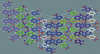Figure 3 Twin domains viewed down the monoclinic b-axis, with alternate layers colored in white, blue, green and magenta. The layers have a width of two hydrogen-bonded molecules (see Fig. 2) and have hydro­phobic faces. The second lattice (left) has a row of molecules in the blue layer in common with that of the white layer in the first lattice (right). The second domain is generated by a twofold rotation around c and a shift over 1/2a. The pseudo-orthorhombic unit cell is shown in red. The hydro­phobic interactions between the layers are almost completely conserved across the twin interface.

As a consequence of the twin obliquity ω = 0.743 (3)°, reflections are split in reciprocal space. This can be seen in simulated precession photographs that were generated with the program PRECESSION in the EVAL package. In the 0th layers, this mainly affects layer h0l (Fig. 4). In the layers, hk0 and 0kl reflections remain nearly unaffected.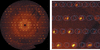Figure 4 Left: simulated precession photograph in the h0l plane of (I) up to a resolution of 0.9 (Å). The reconstruction is based on seven scans with a total of 3324 raw images. Right: zoomed image, is from the yellow square in the left image. White circles are the predicted impacts for the first twin component, blue circles for the second.

### References

This is an open-access article distributed under the terms of the Creative Commons Attribution (CC-BY) Licence, which permits unrestricted use, distribution, and reproduction in any medium, provided the original authors and source are cited.IUCrDATA
ISSN: 2414-3146